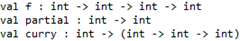# Currying and Partial Applications in F#, Javascript and C#

In my last post I explained the difference between the techniques of Currying and Partial Application, following on where there let me show you how you might apply these two techniques in F#, Javascript and C#.

#### F#

Starting with F#, being a functional language it makes currying and partial application dead easy (considering that they are primarily function programming concepts). Take for example, a simple function f which takes 3 parameters:

```// base function f
let f a1 a2 a3 = a1 + a2 + a3

// partial function fixes two of the parameters
let partial a1 = f a1 5 10

// currying creates a china of functions each taking one parameter
let curry a1 =
// create the next function in the chain which takes another parameter
// and returns another function
let curry' a2 =
// the inner most function gives you the full application
// of the original function
let curry'' a3 = f a1 a2 a3
curry''
curry'
```

From the method signatures you can see how the result of the two approaches differs:To use the partially applied function partial:

```partial 1 // returns 16
```

clearly, this is equivalent to calling

```f 1 5 10 // returns 16
```

seeing as the partial function has fixed a2 and a3 to 5 and 10 respectively.

With the curry function, however, it’s slightly more interesting:

```((curry 1) 5) 10 // returns 16
```

this way of invoking the chained function in turn is in fact the same as:

```curry 1 5 10 // returns 16
```

#### Javascript

Despite being considered an object-oriented language, Javascript shares more than a handful of similarities with function languages, take its dynamic, loose type system, or the ability to treat function as objects, for instance. It’s therefore of little surprise to see that you can implement currying in Javascript with ease.

Here’s the example I used in the last post:

```// original function that takes three parameters a1, a2 and a3
function f(a1, a2, a3) {
return a1 + a2 + a3;
}

// curried function which takes one parameter at a time
function curry(a1) {
// each stage takes another parameter and get you closer to the
// full application of f
return function (a2) {
// but only with the inner most function do you actually get
// the return value you wanted
return function (a3) {
return f(a1, a2, a3);
};
};
}

// partial applied function which takes one parameter and fixes
// the other 2
function partial(a1) {
// a partially applied function of the original function f can
// get you the full application straight away
return f(a1, 5, 10);
}

curry(1)(5)(10); // returns 16
partial(1); // returns 16
```

#### C#

Yes, it’s possible! Here’s a simple example of how using the Func delegate:

```// base function f
Func<int, int, int, int> f = ( a1, a2, a3 ) => a1 + a2 + a3;

// note that partial is a reserved keyword in C#
Func<int, int> partialf = a1 => f(a1, 5, 10);

Func<int, Func<int, Func<int, int>>> curryf = a1 => a2 => a3 => f(a1, a2, a3);

f(1, 5, 10);  // returns 16
partialf(1);  // returns 16
curryf(1)(5)(10);  // returns 16
```

#### Parting thoughts…

There is one important language feature which all three languages support, which is what enables us to apply both currying and partial application so effortlessly – closure. If you’re not familiar with the concept of closure, it’s definitely worth spending a bit of time familiarise yourself with it as it’s a very powerful (albeit often overlooked) language feature.

#### References:

John Resig’s post on currying in JavaScript

Angus Croll – Curry: cooking up tastier functions

Angus Croll – partial: curry’s flashy cousin

Olivier Steel – Functional Javascript

SO question – what is the difference between currying and partial application

Lambda the Ultimate – Currying != Generalized Partial Application?!

Good blog posts which explains the differences between Currying and Partial Application

Did it with .Net – The Art of Currying

### 2 thoughts on “Currying and Partial Applications in F#, Javascript and C#”

1.I found some pretty cool curry examples on the official jPaq site: http://jpaq.org/examples/7 . These examples show how you can use just one line of code to accomplish a lot with pseudo-functional programming.